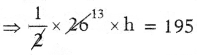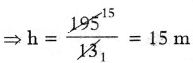SCERT AP 7th Class Maths Solutions Pdf Chapter 11 Area of Plane Figures Ex 11.1 Textbook Exercise Questions and Answers.

## AP State Syllabus 7th Class Maths Solutions 11th Lesson Area of Plane Figures Ex 11.1

Question 1.
Calculate the area of the following triangles given below :
(a)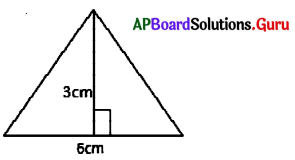From the figure, base = 6 cm
Height = 3 cm
Area of the triangle = $$\frac{1}{2}$$ ∙ b ∙ h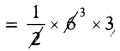= 9 sq.cm(b)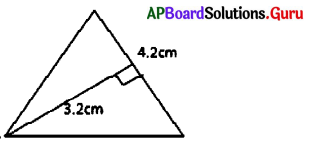From the figure, base = 4.2 cm
Height = 3.2 cm
Area of the triangle = $$\frac{1}{2}$$ ∙ b ∙ h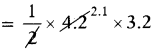= 6.72 sq.cm

(a)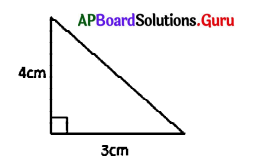From the figure, base = 3 cm
Height = 4 cm
Area of the triangle = $$\frac{1}{2}$$ ∙ b ∙ h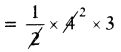= 6 sq.cm

(a)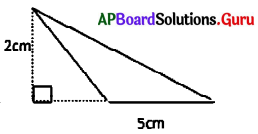From the figure, base = 5 cm
Height = 2 cm
Area of the triangle = $$\frac{1}{2}$$ ∙ b ∙ h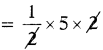= 5 sq.cmQuestion 2.
Find the area of triangle with base 3.8 cm and height 4.6 cm.
Given base of the triangle b = 3.8 cm
Height of the triangle h = 4.6 cm
Area of the triangle = $$\frac{1}{2}$$ ∙ b ∙ h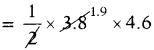∴ Area of the triangle = 8.74 sq.cm.

Question 3.
The surface area of a triangular shape window is 24 sq.m, and height is 6m., then find the base of the triangle, if the cost of glass fitting per sqm is ₹ 250, then find total cost of glass fitting for window.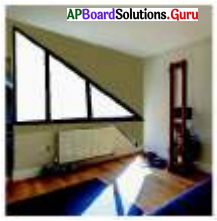Let,
base of the triangular window = b m
Height of the triangular window h= 6m
Area of triangular window = $$\frac{1}{2}$$ ∙ b ∙ h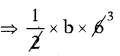= 24 sq.cm
⇒ 3b = 24
⇒ b = $$\frac{24}{3}$$
= 8
∴ Base of the triangular window = 8m
Cost of glass fitting per sq.m = ₹ 250
Cost of glass fitting per 24 sq.m = 250 × 24
∴ Total cost of glass fitting for window = ₹ 6000/-Question 4.
A traffic signal plate in the shape of triangle is having base 20 cm, height 15 cm. Find the area of the triangle. If the cost of painting is ₹ 2 per Sq.cm, then find the total cost for painting the signal board on one side.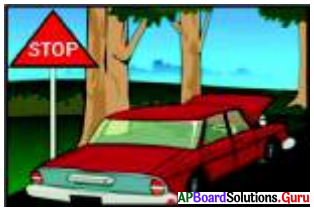Given the base of the signal plate b = 20 cm
Height of the signal plate h = 15 cm
Area of the signal plate = $$\frac{1}{2}$$ ∙ b ∙ h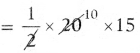= 150 sq.cm
∴ Area of the signal plate = 150 sq.cm
Cost of painting per sq.cm = ₹ 2
Cost of painting for 150 sq.cm = 150 × 2
∴ Total cost for painting the signal board = ₹ 300

Question 5.
find the area of triangular shaped wall painting whose base is 24m, height is 38m. Find the area of the triangle, if the cost of painting is ₹ 50 per Sq. m. and also find the total cost of painting.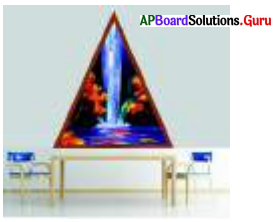Given base of the triangular wall painting = 24 m Height = 38 m
Area of wall painting = $$\frac{1}{2}$$ ∙ b ∙ h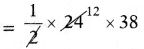= 456 sq.m.
Cost of painting per 1 sq.m = ₹ 50
Cost of painting for 456 sq.m = 456 × 50
∴ Total cost for painting per 456 sq.m = ₹ 22,800Question 6.
The area of triangle shaped elevation of a house is 195 Sq.m. Its base is 26m. Find the height of elevation. Find the total cost of cementing, if the cost of cementing per Sq.m, is ₹ 250.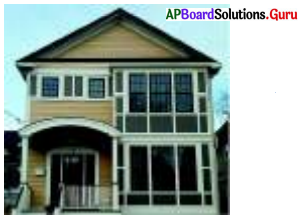= $$\frac{1}{2}$$ ∙ b ∙ h